### IMO Shortlist 2012 problem A1

Kvaliteta:
Avg: 2.5
Težina:
Avg: 6.0
Find all functions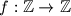$f:\mathbb Z\rightarrow \mathbb Z$ such that, for all integers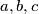$a,b,c$ that satisfy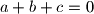$a+b+c=0$, the following equality holds: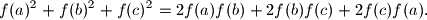(Here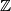$\mathbb{Z}$ denotes the set of integers.)

Proposed by Liam Baker, South Africa
Source: Međunarodna matematička olimpijada, shortlist 2012